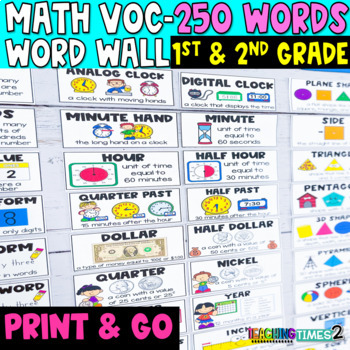# Math Vocabulary Word Wall Cards - 1st & 2nd Grade

Rated 5 out of 5, based on 2 reviews
2 Ratings;
1st - 2nd, Homeschool
Subjects
Resource Type
Standards
Formats Included
• Zip
Pages
Report this resource to TPT

### Description

Math Word Wall Grade 1 & 2 Vocabulary Cards: Help your 1st or 2nd-grade students remember these essential math vocabulary terms with this visual math word wall while brightening up your classroom at the same time!

MATH HEADERS INCLUDED: GEOMETRY, MEASUREMENT & DATA, OPERATIONS & ALGEBRAIC THINKING, NUMBERS & OPERATIONS in BASE 10

Math vocabulary words included:

MEASUREMENT & DATA

• analog clock, digital clock, second, o'clock
• minute hand, hour hand, hour, half-hour
• half past, quarter past, calendar, year, week
• month, schedule, day, A.M., P.M.
• cent, cents, penny, nickel, dime
• quarter, half dollar, dollar sign, dollar
• decimal point, spend, save
• measuring tools, length, unit, shortest
• longest, centimeter, width, inch
• foot, yard, meter, height, area, perimeter
• measure, cup, pint, quart, gallon
• liter, pound, gram, kilogram
• capacity, weight, balance scale
• lighter, heavier, attribute, temperature
• degrees, Fahrenheit, Celsius
• estimate, tally marks, data, bar graph
• pictograph, line plot, certain
• likely, unlikely, equally likely, impossible

NUMBERS & OPERATIONS IN BASE TEN-

• digit, ones, tens, hundreds, thousands
• place value, place value chart, standard form
• expanded form, word form, number word
• greatest, least, pair, odd, even
• before, between, after, skip counting
• pattern, ordinals, mental math, predict
• 1 more, 1 less, 10 more, 10 less, next ten
• 100 more, 100 less, compose, decompose
• open number line, number line
• part part whole, number bonds, regroup
• greater than, less than, equal to
• ten frame, make 10, repeat

OPERATIONS & ALGEBRAIC THINKING-

• less than, greater than, rename, equation
• greatest, least, before, between, after
• near double, doubles plus 1, inside, outside
• in all, whole, equals, plus, join, add
• subtraction, minus, missing part, difference
• subtraction sentence, equals sign, related facts
• fact family, input, output, separate
• three-digit number, increase, decrease
• multiplication sentence, diagonal, column
• array, minuend, compare
• equal parts, regroup, more
• fewer, multiply, product
• number sentence, vertical, horizontal
• divide, count back, count on
• equal share, subtrahend, number path

GEOMETRY-

• 2D shape, plane shape, corner
• side, square, triangle
• rectangle, circle, oval
• star, hexagon, octagon
• pentagon, polygon, rhombus
• 3D shape, cube, sphere
• pyramid, solid figure, cylinder
• cone, rectangular prism, vertices
• edge, face, flat surface
• sort, set, venn diagram
• slide, turn, flip
• translation, reflection, rotation
• symmetry, line of symmetry, congruent
• unequal, equal, fraction
• whole, part, halves
• thirds, fourths, quarters
• partition, numerator, denominator
• right angle, acute angle, obtuse angle
• row, column

▼▼▼▼▼▼▼▼▼▼▼▼▼▼▼▼▼▼▼▼▼▼▼▼▼▼▼▼▼▼▼▼▼▼▼▼▼▼▼▼▼▼▼▼▼

If you would like to get updates on NEW and CURRENT resources....

FOLLOW US on TEACHERS PAY TEACHERS!

FOLLOW the TEACHING TIMES 2 BLOG!

Thanks and remember to keep learning FUN!! --Kristy & Misty ❤

▼▼▼▼▼▼▼▼▼▼▼▼▼▼▼▼▼▼▼▼▼▼▼▼▼▼▼▼▼▼▼▼▼▼▼▼▼▼▼▼▼▼▼▼▼

Permission to copy for single classroom use only.

Electronic distribution is limited to single classroom use only.

Total Pages
N/A
Teaching Duration
1 Year
Report this resource to TPT
Reported resources will be reviewed by our team. Report this resource to let us know if this resource violates TPT’s content guidelines.

### Standards

to see state-specific standards (only available in the US).
Order three objects by length; compare the lengths of two objects indirectly by using a third object.
Express the length of an object as a whole number of length units, by laying multiple copies of a shorter object (the length unit) end to end; understand that the length measurement of an object is the number of same-size length units that span it with no gaps or overlaps.
Tell and write time in hours and half-hours using analog and digital clocks.
Organize, represent, and interpret data with up to three categories; ask and answer questions about the total number of data points, how many in each category, and how many more or less are in one category than in another.
Measure the length of an object by selecting and using appropriate tools such as rulers, yardsticks, meter sticks, and measuring tapes.